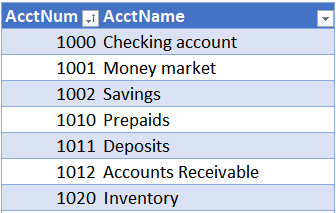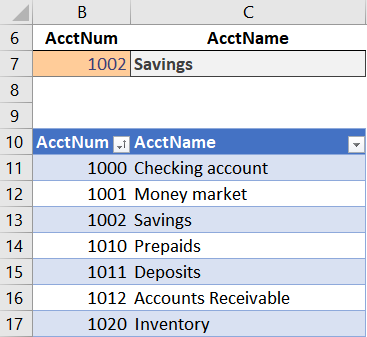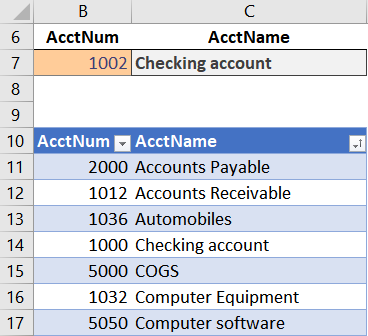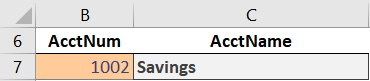# VLOOKUP Hack #1: Sort OrderVLOOKUP is one of the most popular Excel functions. It can do amazing things and help save time. But, there are some really cool hacks that make it even better. This is the first post in a series of VLOOKUP Hacks.

Hope you enjoy 🙂

## Issue

VLOOKUP Hack #1 helps address the sort issue. Sort issue? Yes, and the sort issue has confuzzled many an Excel user over the years.

I’ve included a short video demonstration as well as a detailed narrative below for reference.

## Detailed Narrative

Let’s say we wanted to use VLOOKUP to retrieve an account name based on the account number from a chart of accounts, as shown below.Assuming the lookup value is B7, the lookup range is Table1, and the account name is in the second column, we could use something like this in C7:

`=VLOOKUP(B7,Table1,2)`

When the table is sorted in ascending order by the lookup column, the AcctNum column, you get the expected result. For example, if the AcctNum is 1002, the VLOOKUP function above returns the expected account name, Savings, as shown in C7 below.But, if the table get sorted by AcctName instead, we get an unexpected result, as shown below.Wait, what? 1002 is not Checking account, yet, that is what VLOOKUP returns … is it broken? Is the formula wrong? What’s up? Do I have to sort by the lookup column? What is going on here?

These questions all bring us to our first hack.

## Hack

So, the VLOOKUP formula above is written like this:

`=VLOOKUP(B7,Table1,2)`

You will notice that 3 arguments are defined, B7, Table1, and 2.

But, here is the hack: there is an optional 4th argument!

When the 4th argument is omitted, as in the formula above, it takes on a default value. So, we need to unpack this mysterious 4th argument to understand what is happening.

The 4th argument controls the behavior of VLOOKUP in a couple of key ways, including, as you may suspect, the sorting requirement. Officially, the 4th argument is called the range_lookup argument. Practically, it tells Excel whether you are looking for an exact matching value, or, a value between a range of values. It is a Boolean argument, so it expects a TRUE or FALSE value. (Alternatively, you can use 0 instead of FALSE and any non-zero number instead of TRUE.) TRUE means you are looking for a value between a range of values, and FALSE means you are looking for an exact matching value. I’ll expand this idea more in the next post, but for now, I want to stay focused on the sort issue.

When the 4th argument is TRUE, the data must be sorted in ascending order to return an accurate result. However, when the 4th argument is FALSE, the data need not be sorted…any order is fine.

So, when the 4th argument is TRUE, you may get an unexpected result if the data is not sorted in ascending order by the first column in the range. And, that is exactly what we saw above. We got an unexpected result. Instead of 1002 returning Savings, it returned Checking account. But, if we inspect the formula, we can see that we did not specify TRUE or FALSE for the 4th argument. We simply omitted it.

Here is the key: when omitted, the 4th argument is TRUE.

That means that when we write a VLOOKUP, and don’t specify the 4th argument, it defaults to TRUE. That means, sort order matters! When the data is not sorted in ascending order by the first column, you may get unexpected results.

So, we can resolve the issue by using FALSE (or 0) as the 4th argument, as shown below.

`=VLOOKUP(B7,Table1,2,FALSE)`

With that update to our formula, it returns Savings, as expected:So, remember this: when the 4th argument is TRUE or omitted, the lookup range must be sorted in ascending order by the first (lookup) column to return an accurate result. But, when the 4th argument is FALSE (or 0), the data does not need to be sorted.

In the next post, we’ll examine the 4th argument in more detail. It allows us to perform some really fun lookups!

Get a quick email notice when a new Excel article is available

• This field is for validation purposes and should be left unchanged.1.SusanM

Thanks for putting a spotlight on this issue! In the past I’ve sorted the table, but ran into the issue when I added some rows & didn’t re-sort. Now I know how to fix the formula, that’s awesome.

1.Jeff Lenning Post author

Welcome 🙂

2.Patrick Westrick

Thanks Jeff, I didn’t know that and it’s always great to learn new things.

1.Jeff Lenning Post author

Welcome … I learn new things about Excel all the time 🙂

3.Mark

Jeff, thanks very much. This repeats something I’ve found in VLookups.
Using “False” in that 4th step seems to yield a cleaner search and/or
sort result. It’s helpful to confirm that because it keeps the search simple
and basic. Mark Ferguson

4.Dave Slomer

Excel 2007 (despite no online help from Microsoft, which is why I’m here) still works well enough for me… or does it…

When I add a 4th argument to LOOKUP as shown
=LOOKUP(MID(G25,7,9),F:F,J:J,FALSE)
I get error: “You have entered too many arguments for this function”
so I assume this 2017 article only applies to Excel 2010 and beyond.

Too bad. Great hack in some versions, I guess….

1.Jeff Lenning Post author
5.Eddie